RUSSIAN JOURNAL OF EARTH SCIENCES, VOL. 20, ES6014, doi:10.2205/2020ES000752, 2020

# FDPS algorithm in stability assessment of the Earth's crust structural tectonic blocks

S. M. Agayan1, V. N. Tatarinov1,2, A. D. Gvishiani1,2, Sh. R. Bogoutdinov1,2, I. O. Belov1

1The Geophysical Center, Russian Academy of Sciences, Moscow, Russia

2Schmidt Joint Institute of Physics of the Earth, Russian Academy of Sciences, Moscow, Russia

### Abstract

The paper presents the structure of a new original FDPS (Functional Discrete Perfect Sets) algorithm used to filter and arrange the layers of the geospatial data into the homogenous groups and identify dense homogenous condensations. The latter may be related to the deep zones of dynamic instability in the upper part of the Earth's crust. Synthetic and real examples of this algorithm's usage are presented, demonstrating its capabilities as part of the system analysis of the geological environment stability in the area of construction of a deep disposal site for high-level radioactive waste. Testing the algorithm allowed us to identify the most stable blocks, thereby demonstrating its usage value. This shows the necessity of further development and use of the FDPS algorithm.

### Introduction

At present, an underground research laboratory (URL) is being created in the granite-gneiss rocks of the Nizne-Kansk Massif (Krasnoyarsk Territory) to assess and substantiate the geoecological safety of disposal of high-level radioactive waste (HLRW). In international and Russian documents regulating safety of HLRW management, the main message is the idea that the main barrier in the way of spreading of radionuclides is the geological environment. The engineering barriers for HLRW with the half-life of more than 10 thousand years are secondary.

The selection of the part of the Earth's crust suitable for HLRW disposal is based on the analysis of properties, phenomena and processes affecting preservation of the insulating properties of the rocks of the structural tectonic block (STB) containing HLRW. The complexity of solving this multidisciplinary problem is related to the extreme heterogeneity of the upper part of the Earth's crust caused by a structural-tectonic disturbance (lineaments, fractures, large cracks, etc.) and tectonic movements of various hierarchical levels (differentiated movements along the fracture, tectonic creep, seismicity, etc.).

The Nizne-Kansk Massif is located in the aureole of the largest regional tectonic structures – the folded structure of the Yenisei Ridge, the epi-Hercynian West Siberian platform, the ancient Siberian platform and the young Altay-Sayan earthquake-prone region. The force interaction of these structures specifies the current stress-strain state of the region.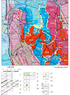Figure 1

The southern part of the Yenisei Ridge (Figure 1) since the end of the Pleiocene ($1.5\pm 0.5$ mln years) has been experiencing a slow uplift, the total amplitude of which is estimated at 400-500 m, and the average velocity according to the geological data makes 0.2-0.3 mm/year [Anderson et al., 2011; Belov et al., 2007; Lobatskaya, 2005].

As applied to the problem of the HLRW disposal in geological formations, an important term was introduced – "stability of the structural-tectonic block" [Tatarinov et al., 2014a; Gvishiani et al., 2019a]. It is understood as the capacity of the block to maintain or change its properties and state under the natural and anthropogenic influences within the range that will not lead to the loss of insulating properties of the rock mass and release of radionuclides outside the sanitary zone. This is defined by the time interval equal to the period of the HLRW radiobiological hazard.

The structural tectonic block is the system with distributed parameters depending on the time and coordinates of observation points. Their anomalous values (potentially dangerous for preservation of insulating properties of the rocks) are represented in the features (morphology) of distribution of the geological, geophysical, geochemical and other characteristics and the Earth's surface relief, most often in the form of linearly elongated areas, the so-called geodynamic zones. It is believed that geodynamic zones are responsible for:

• formation of local zones of concentration of stress fields, initiating the process of rock destruction in the form of fast seismic processes or tectonic creep;
• modern vertical and horizontal movements of the Earth's crust;
• destruction of the rock mass and an increase in its permeability and porosity for the groundwaters.

Their identification is the most significant task of geodynamic zoning [Morozov et al., 2008; Petukhov et al., 1999; Tatarinov et al., 2014b].

In most cases, it is almost impossible to visually identify unstable zones in the maps, especially based on the set of features. It is characteristic for low-level platform areas (in fact, to which the Nizne-Kansk Massif belongs) or regions with a thick sedimentary cover. For such cases, based on the methods and algorithms of discrete mathematical analysis (DMA) [Agayan et al., 2018; Gvishiani et al., 2019b] within the framework of the Russian Science Foundation project no. 18-17-00241, a measure of geodynamic safety was constructed considering interaction of the geodynamics and morphological features of distribution of geological and geophysical parameters (including a digital terrain model, results of GNSS-observations, geophysical fields, etc.) [Gvishiani et al., 2019a; Gvishiani et al., 2020]. In accordance with the values of this measure, the studied area is ranked into relatively unstable (conditionally dangerous) and stable (conditionally safe) structural blocks. It is required to create an algorithm of adequate system analysis to identify them. An original algorithm FDPS (Functional Discrete Perfect Sets) is developed and applied in the paper for this purpose. The first results of its use in the considered region are stated in this paper.

### 1 Construction FDPS Algorithm

The search for anomalies in the fields of geophysical data [Mikhailov et al., 2003; Zlotnicki et al., 2005; Soloviev et al., 2012], identification of the places of possible occurrence of significant earthquakes [Gvishiani et al., 2016; Gvishiani et al., 2017] and other tasks related to the problems of natural risk bring a researcher (an expert) to the need to assess $\nu(x)\in [0,1]$ the nodes $x$ of the finite grid $X$ based on the measurements carried out in them. The assessment $\nu$ is required to rank the nodes in $X$ and select the subsets $B(X,\nu)$ of the best nodes among them.

In general, the assessment $\nu$ inherits stochasticity of the measurements underlying it. That's why, the selection according to the level $\alpha$ as to $\nu$

\begin{eqnarray*} B(X,\nu) = \left\lbrace x\in X: \, \nu(x)\ge \alpha \right\rbrace \end{eqnarray*}

is unstable: a "good node" $x$ ($\nu(x)\ge \alpha$) may be surrounded with "bad nodes" $x$ ($\nu(x)< \alpha$), and that's why it is not considered further. And vice versa, a "bad node" may be surrounded with "good nodes" and will be used in further work.

The aim of this work is to form a selection $B(X,\nu)$ in presence of the metric structure on $X$ using the Discrete Perfect Sets topological filtering algorithm developed in the frames of discrete mathematical analysis (DMA) and its application to the HLRW disposal problem.

This selection will represent a set of regions connected in $X$, in which the majority of nodes are "good".

#### 1.1 Discrete Perfect Sets

Let $X$ be the finite set, and $A$, $B$, . . . and $x$, $y$, . . . – its subsets and points, respectively.

Definition 1 Let's call a mapping of $2^X\times X$ to the fragment $[0, 1]$, increasing by the first argument, the density $P$ on the set $X$:

\begin{eqnarray*} \begin{array}{c} P(A, x) = P_A(x) \forall x \in X \colon A \subseteq B \Rightarrow P_A(x) \leq P_B(x). \end{array} \end{eqnarray*}

$P_A(x)$ is the density of the subset $A$ in the point $x$.

For the density $P$ given on $X$, the subset $A$ and the level $\alpha\in [0, 1]$, let's construct a sequence of $\alpha$-$n$-hulls of the subset $A$ in the set $X$ according to the density $P$:

\begin{eqnarray*} A^1 = x \in X \colon P_A(x) \geq \alpha, &. . . A^n = x \in X \colon P_{A \cup A^{n - 1}}(x) \geq \alpha, &. . . \end{eqnarray*}

Induction by $n$ using the increasing monotonicity $P$ specifies

Statement 1 $A^1 \subseteq \dots \subseteq A^n \subseteq \dots .$

Because of finiteness of the set $X$, in the non-decreasing and bounded sequence of $\alpha$-$n$-hulls, starting from some number $n^*$, stabilization occurs:

Definition 2 Let's call the set $A^{n^*}$ $\alpha$-$\infty$-hull of the subset $A$ and designate through $A^{\infty}$.

The set $A^{\infty}$ is semi-variant: its first density hull $(A^{\infty})^1$ does not fall beyond the set $A^{\infty}$.

Statement 2 $A^{\infty}$ contains its $\alpha$-hull by the density:

\begin{eqnarray*} (A^{\infty})^1 \subseteq A^{\infty} . \end{eqnarray*}

P r o o f by contradiction. Let us use the finite representation $A^{\infty}$: $A^{\infty}=A^{n^*}$. If $P_{A^{n^*}} (x)\ge \alpha$ and $x \notin A^{n^*}$, then $P_{A \cup A^{n^*}} (x)\ge P_{A^{n^*}} (x)\ge \alpha \Rightarrow$ $x \in A^{(n^*+1)}$ and therefore $A^{n^*} \subset A^{(n^*+1)}$. However, based on the condition $A^{n^*} = A^{(n^*+1)}$. The obtained contradiction proves the statement.

Form this it immediately follows that for a set the series of its $\alpha$-$n$-hulls is constant.

Consequence

\begin{eqnarray*} A^{\infty})^n = (A^{\infty})^1 \forall \; n \ge 2. \end{eqnarray*}

P r o o f. It is stated above that $(A^{\infty})^1 \subseteq A^{\infty}$ therefore,

\begin{eqnarray*} \begin{split} (A^{\infty})^2 &= \left\lbrace x\in X: P_{A^{\infty} \cup (A^{\infty})^1} (x) \right. = &= \left. P_{A^{\infty}} (x)\ge \alpha \right\rbrace = (A^{\infty})^1 \end{split} \end{eqnarray*}

and so on.

Let's designate $\alpha$-$\infty$-hull for $A^{\infty}$ through $A^{2 \infty}$. We have:

\begin{eqnarray*} A^{2 \infty} = (A^{\infty})^{\infty} = (A^{\infty})^1 \subseteq A^{\infty} . \end{eqnarray*}

Sequentially constructing the $\alpha$-$\infty$-hulls based on the density $P$, we obtain the following scheme:

\begin{eqnarray*} A \rightarrow A^1 \subseteq \dots \subseteq A^{\infty} A^{\infty} \supseteq (A^{\infty})^1 = A^{2 \infty} \dots A^{m \infty} \supseteq (A^{m \infty})^1 = A^{(m + 1) \infty} \dots \end{eqnarray*}

Because of $X$ finiteness in a non-increasing sequence

\begin{eqnarray*} A^{\infty} \supseteq \dots \supseteq A^{m \infty} \supseteq \dots , \end{eqnarray*}

starting with some number $m^*$, stabilization occurs:

\begin{eqnarray*} A^{\infty} \supset \dots \supset A^{m^* \infty} = A^{(m^* + 1) \infty} = \dots . \end{eqnarray*}

Definition 3 Let's designate the set $A^{m^* \infty}$ through $A(\alpha)$.

The process of constructing $A(\alpha)$ has a stage of increasing from $A^1$ to $A^{\infty}$ and a stage of decreasing from $A^{\infty}$ to $A(\alpha)$:

\begin{eqnarray} \tag*{(1)} A &\rightarrow A^1 \subset \dots \subset A^{n^*}= \end{eqnarray} \begin{eqnarray*} = A^{\infty} \supseteq \dots \supseteq A^{m^* \infty} = A(\alpha). \end{eqnarray*}

Statement 3 $A(\alpha)$ coincides with its $\alpha$-hull.

P r o o f. It follows from the following results:

\begin{eqnarray*} A(\alpha) = A^{n^* \infty} = A^{(n^* + 1) \infty} = (A^{n^* \infty})^1 = (A(\alpha))^1 . \end{eqnarray*}

Informally, this statement means that the set $A(\alpha)$ consists exactly of those points where its density is more than $\alpha$ or equal to it:

\begin{eqnarray*} A(\alpha) = \left\lbrace x\in X: P_{A(\alpha)} (x)\ge \alpha \right\rbrace . \end{eqnarray*}

In all complement points, the density $P_{A(\alpha)}$ is less than $\alpha$:

\begin{eqnarray*} \overline{A(\alpha)} = \left\lbrace x\in X: P_{A(\alpha)} (x)< \alpha \right\rbrace . \end{eqnarray*}

It follows particularly from here that the process of transition from $A(\alpha)$ to $A(\alpha)(\alpha)$ is constant and therefore $A(\alpha)=A(\alpha)(\alpha)$.

Informal interpretation of $A(\alpha)$. Let's interpret the density $P_A(x)$ as a measure of limit of the point $x$ for the set $A$. The point $x$ with a sufficiently high density

\begin{eqnarray*} P_A(x) \geq \alpha \end{eqnarray*}

are considered to be limit for $A$. The set $A^{\infty}$ includes all its $\alpha$-limit points from $X$ is closed in this respect. The set $A(\alpha)$, included into $A^{\infty}$, coincides with the set of its $\alpha$-limit points from $X$ and is perfect in this respect.

#### 1.2 Complete Discrete Perfect Sets Algorithm

Definition 4 The construction process for the set $A$ in the universe $X$ based on the density $P$ is called, complete Discrete Perfect Sets algorithm and is designated through $\mathbb{DPS}$:

\begin{eqnarray*} \mathbb{DPS} (\cdot) =\mathbb{DPS}(\cdot | X, P, \alpha): 2^X \rightarrow 2^X. \end{eqnarray*}

It is specified above that the algorithm $\mathbb{DPS}$ is idempotent $(\mathbb{DPS}^2 = \mathbb{DPS})$. The subsets which are fixed in respect to it are called $\alpha$-perfect sets of ($\alpha$-$\mathbb{DPS}$-sets) in $X$:

\begin{equation} \tag*{(2)} \begin{array}{l} A=\alpha \text{-} \mathbb{DPS} \text{-sets in } X \Leftrightarrow \mathbb{DPS}(\cdot | X, P, \alpha) = A \Leftrightarrow A = \left\lbrace x\in X: P_{A(\alpha)} (x)\ge \alpha \right\rbrace \end{array} \end{equation}

In general, as it is stated above (1), the $\mathbb{DPS}$ algorithm has two stages: increasing

\begin{eqnarray*} A^n \uparrow A^{\infty} \Leftrightarrow A\rightarrow A^1 \subseteq \dots = A^{\infty} \end{eqnarray*}

and decreasing

\begin{eqnarray*} A^{m \infty} \downarrow A^{\infty} \Leftrightarrow A^{\infty} \supseteq A^{2 \infty} \supseteq \dots A(\alpha) . \end{eqnarray*}

There are situations when the algorithm $\mathbb{DPS}$ "works faster" and has no more than one stage. A trivial case is examined in (3): $\mathbb{DPS}$ is fixed at $A \equiv$ zero algorithm stages $\mathbb{DPS}$ at $A$ – $\alpha$-perfect. Let's study $\mathbb{DPS}$ with one stage.

Increasing $\mathbb{DPS}$. There is only an increasing stage $A^n \uparrow A^{\infty}$ available, therefore, the set $A$ is $\alpha$-perfect.

Statement 4 Sufficient condition of increasing $\mathbb{DPS}$: $A \subseteq A^1 \equiv$ any point in $A$ is $\alpha$-limit for it.

P r o o f. In this case $A\subseteq A^n \; \forall \; n\ge 1$ and therefore

\begin{eqnarray*} A^{n+1} = \left\lbrace x\in X: P_{A\cup A^n} (x)\ge \alpha \right\rbrace = \left\lbrace x\in X: P_{A^n} (x)\ge \alpha \right\rbrace = (A^n)^1. \end{eqnarray*}

If $A^{\infty} = A^{n^*}$, then$A^{\infty} = A^{n^*+1}= (A^{n^*})^1 = (A^{\infty})^1$ which means $\alpha$-perfection of $A^{\infty}$ (3).

Decreasing $\mathbb{DPS}$. There is only a decreasing stage $A^{m \infty} \downarrow A(\alpha)$ available. There is no increasing stage, therefore, $A^1 = A^{\infty}$. Let's give a simple and effective criterion of this situation:

Statement 5 $A^1 = A^{\infty} \Leftrightarrow A^1 = A^2$.

P r o o f. The necessity $A^1 = A^{\infty} \Rightarrow A^1=A^2$ follows from inclusions $A^1 \subseteq A^2 \subseteq A^{\infty}$.

Sufficiency $A^1=A^2 \Rightarrow A^1 = A^{\infty}$. In this case,

\begin{eqnarray*} A^3 = \left\lbrace x\in X: P_{A\cup A^2} (x)\ge \alpha \right\rbrace = \left\lbrace x\in X: P_{A\cup A^1} (x)\ge \alpha \right\rbrace = A^2 . \end{eqnarray*}

Similarly, $A^4=A^3, \dots, A^{n+1}=A^n$ and so on, that's why, $A^1 = A^{\infty}$.

Example Assume that $A^1 \subseteq A$, then

\begin{eqnarray*} A^2 = \left\lbrace x\in X: P_{A\cup A^1} (x)\ge \alpha \right\rbrace = \left\lbrace x\in X: P_{A} (x)\ge \alpha \right\rbrace = A^1 \end{eqnarray*}

The condition $X^1 \subseteq X$ is evidently fulfilled, that's why throughout the total space $X$ the algorithm $\mathbb{DPS}$ is always decreasing. Let's present it in full because of its great practical importance:

\begin{eqnarray*} \begin{split} X(\alpha) =& \cap X^n (\alpha), X^n(\alpha) = & \left\lbrace x\in X: P_{X_{(\alpha)}^{n-1}} (x) \ge \alpha \right\rbrace , &n\ge 1, X^0 (\alpha) = X. \end{split} \end{eqnarray*}

The $\mathbb{DPS}$ algorithm constructs for each subset of the original space its "perfect shell", which we consider to be a cluster. Thus, $\mathbb{DPS}$ answers for each subset the question about the closest and naturally related cluster. The complete $\mathbb{DPS}$ is needed to accurately scrutinize the effect of an original subset on its surrounding complement.

In a sense, a simple $DPS$ is the opposite of a complete one, building a perfect envelope for all space. This is the largest perfect set. If there is a metric in the original space, $DPS$ splits it into connected pieces that are of interest to us and which we consider to be clusters.

#### 1.3 Simple Discrete Perfect Sets Algorithm

The first part of the simple Discrete Perfect Sets algorithm ($DPS$) consists in the transition $X\rightarrow X(\alpha)$, i.e., in cutting out from the whole set $X$ of its $\alpha$-perfect part $X(\alpha)$ with respect to the densities requiring the presence of the metric $d$ on $X$. Let's describe two structures of such density. The first of them is called "Number of points" and the set-theoretic algorithm SDPS is related to it. The second is called "Average weight" and the functional algorithm FDPS is related to it.

Number of points. The density depends on the proximity radius $r$ and the parameter $q\ge 0$. A ball with the center at $x$ of radius $r$ is considered for each point $x$ from the set $X$:

\begin{eqnarray*} D(x,r) = \left\lbrace y\in X: d(x,y)\le r \right\rbrace . \end{eqnarray*}

The sum of points of the set $X$ in it is calculated for each ball taking into account the distance from the point to the ball center:

\begin{eqnarray*} N_X (x,r) = \sum _{y\in D(x,r)} \left(1 - \frac{d(x,y)}{r} \right)^q . \end{eqnarray*}

Let's designate the maximum of such sums by all point $x\in X$ through $N(X,r)$:

\begin{eqnarray*} N(x,r) = \underset{x\in X}{\max}N_X(x,r) . \end{eqnarray*}

Also, the sum of points is calculated for each ball considering their distance from the ball points only based on the points of the subset $A\subseteq X$:

\begin{eqnarray*} N_X (X,r) = \sum _{y\in D_A(x,r)} \left(1 - \frac{d(x,y)}{r} \right)^q . \end{eqnarray*}

Here, $D_A (x,r)$ is the intersection of the ball $D(x,r)$ and the subset $A$:

\begin{eqnarray*} D_A (x,r) = D(x,r) \cap A . \end{eqnarray*}

The density of the subset $A\subseteq X$ in the point $x\in X$ is determined as the ratio of the sum of points of the ball in $A$ considering their distance from the center $x$ to the maximum sum of the balls in $X$:

\begin{equation} \tag*{(3)} P_A (x) = \frac{N_A(x,r)}{N(X,r)} \end{equation}

Average Weight. The functional variant of the simple algorithm $DPS$ is related to a special density $P(\nu,r)$ based on the weight function $\nu: X\rightarrow [0,1]$ and localization of the radius $r$:

\begin{equation} \tag*{(4)} P_A(x) = \frac{\sum \nu (\bar{x}): \bar{x}\in D_A(x,r)}{|D(x,r)|} . \end{equation}

The value $\nu(x)$ may be assumed as the weight of the element $x$.

Topological Deviation. It will be recalled that two points $x$ and $y$ in $A\subseteq X$ are called $r$-connected, if there is a chain of $r$-close points $x_0,\dots,x_n$ in $A$ with the beginning $x_0=x$ and the end $x_n=y$. The $r$-connectivity ratio is an equivalence and breaks $A$ into $r$-connectivity components $CA(1),\dots,CA(k^*)$, $k^*=k^*(A)$:

\begin{eqnarray*} A=CA(1)\vee \dots \vee CA(k^*) . \end{eqnarray*}

Statement 6 If $P_A(x)$ of densities is (5) or (6), the components of $r$-connectivity $CX(\alpha)(1),\dots,$ $CX(\alpha)(k^*)$ are $\alpha$-perfect.

P r o o f. Such densities have an $r$-local action: if $d(x,A)>r$, then $P_A(x)=0$. Therefore, for any component of the $r$-connectivity $CX(\alpha)(k)$, the following equality is valid

\begin{eqnarray*} P_{CX(\alpha)(k)}(x) = P_{X(\alpha)}(x) \forall \, x\in CX(\alpha)(k). \end{eqnarray*}

The $\alpha$-perfection $CX(\alpha)(k)$ follows from here and the $\alpha$-perfection of $X(\alpha)$.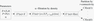Figure 2

Informally, this statement means consistency of $\alpha$-perfection and connectivity, which is the theoretical justification for the second clustering part of the $DPS$. The algorithm flow diagram is given in Figure 2

Let's summarize the above said giving the following.

Definition 5

1. The process of construction for the finite metric space $(X,d)$ based on the $r$-local density $P$ of the $\alpha$-hull $X(\alpha)$ with its subsequent partition into $r$-connected components is called a simple $DPS$ algorithm:
\begin{eqnarray*} DPS = DPS (P, \alpha, r): X\rightarrow 2^{2^X} DPS (X) = CX(\alpha)(1),\dots,CX(\alpha)(k^*); \end{eqnarray*}
1. If the density $P$ has the form (5), the $DPS$ is called set-theoretic (SDPS);
2. If the density $P$ has the form (6), the $DPS$ is called functional (FDPS).

In conclusion, let's turn out attention to the relations of the SDPS algorithm and cluster analysis. For this purpose, let's present a heuristic definition of clusters given by Everitt: "Clusters are `continuous' areas of a (certain) space in related to a higher density of points, separated from other similar areas by the areas with a relatively low density of points" [Everitt, 1980].

Implementation of this definition is more than a traditional cluster analysis [Tou and Gonzalez, 1974], because it involves not only partition of the initial space into clusters, but also its preliminary reduction (filtering) prior to their union.

The SDPS algorithm makes it and, that's why, it represents an algorithm of a new post-clustering stage in the cluster analysis.

#### 1.4 Synthetic examples of use of the SDPS and FDPS algorithms

The "Number of points" density (5) shows the degree of concentration of the space $X$ around each of its nodes $x$. Therefore, the set-theoretic SDPS is focused on the search for condensations and works well in non-homogeneous spaces (irregular grids) $X$.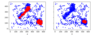Figure 3

Figure 3 shows the work of the SDPS algorithm on an irregular grid with different parameters ($r$, $\alpha$). By varying them, one can get a fairly complete idea of the concentrations in the original space. The given examples illustrate the general property of the dependence of the $DPS$ algorithm on parameters: the smaller the proximity radius and the higher the density level, the stricter the $DPS$ algorithm, and the denser and finer its results.

FDPS algorithm (6) it is focused on the search for the subsets in $X$ with the $r$-locally high exponent of weights $\nu$. It is also capable of working on regular grids, and therefore it successfully complements the SDPS algorithm.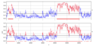Figure 4

Figure 4a shows the work of the FDPS algorithm: the space $X$ in this case is a regular grid on the horizontal axis, the weight $\nu$ of each point $x\in X$ is plotted vertically. The results of the FDPS algorithm are two red segments on the horizontal axis, which serve as the bases of the two most significant stochastic heights on $X$. As you can see from the figure, the FDPS algorithm is stable and does not pay attention to insignificant drops below a given level. This property explains the massiveness of the heights allocated to him. For comparison, Figure 4b shows a classic selection on a grid relative to a given level. This approach, in our opinion, gives numerous weak results located outside the limits of the massive segments distinguished by the algorithms FDPS (Figure 4a).

### 2 Results of Application of the FDPS Algorithm to the Fata of the Nizne-Kansk Massif

The work of the SDPS algorithm for recognizing places of possible occurrence of strong earthquakes has shown its stability. Epicenters of strong earthquakes stably fall into clusters obtained by SDPS in the set of epicenters of all earthquakes. These clusters are in good agreement with the zones allocated by the well-known EPA algorithm [Gvishiani et al., 2016; Gvishiani et al., 2017].

It will be shown below that the FDPS algorithm has the same property in the problem of assessment the stability of structural tectonic blocks of the earth's crust.

In this case, the weight function $\nu$ for it was an integral measure of stability, calculated in the area of the Nizne-Kansk Massif (Krasnoyarsk Territory) and ranking for the safety of nuclear waste disposal: the more the measure is, the safer the object under study is.

Construction of $\nu$ requires combining heterogeneous information from the geological and geophysical parameters and therefore represents a problem of the system analysis. It is solved within the frames of the program for studying the systems of real-valued functions on two-dimensional grids using the fuzzy sets [Gvishiani et al., 2019b] created by the authors.

Its final stage – selection of the connected massif areas that are relatively stably-high – is solved by the FDPS algorithm and in our case represents zones suitable for safe disposal in the Nizne-Kansk Massif.

#### 2.1 Integral Stability Measure

The integral measure of stability $\nu$ is calculated based on the complex of the geological-geophysical parameters. Some of them have a natural character and are related to the relief of the Nizne-Kansk Massif and its system of fractures. The other part represents modeling of the stress-strain state of the Nizne-Kansk massif based on its GNSS observations.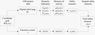Figure 5

As noted above, the theoretical foundations of the approach are represented in the paper [Gvishiani et al., 2019b]. The calculation diagram includes the following stages (Figure 5):

1. "Dynamic indicator" – a primary analysis of the initial geological, geophysical and geomorphological data. Each dynamic indicator is interpreted as a quantitative assessment of one or another property of the initial data.
2. "The measure of activity of the dynamic indicator" – this measure shows the degree of activity of the studied property of the geological environment in the scale $[0,1]$.
3. "The measure of safety of the dynamic index" is an fuzzy negation [Zadeh, 1996] of its measure of activity and characterizes weakness of appearance of the property of this dynamic indicator. Transition to the safety measure means translation of the initial data into the language of fuzzy logic. The safety measures of dynamic indicators are fuzzy structures and, therefore, they can be united in any compositions and quantities using fuzzy logic operations.
4. "Integral safety measure" is an integral combination of safety measures of the dynamic indicators and represents the measure of geodynamic safety of the studied area.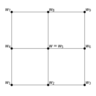Figure 6

The $W$ node grid with dimensions ($250\times 150$) was chosen to implement the methodology in the area of the Nizne-Kansk Massif. Let's call the node $w\in W$ internal, if it is surrounded with eight adjacent nodes of the grid (Figure 6).

Four indicators were calculated in each internal node of the grid characterizing the features of the relief $L_{Re}^1$, $L_{Re}^2$, $\nabla_{Re}$ and the proximity to active fractures – $\rho(\pi, P_k)$. The first two indicators ($L_{Re}^1$, $L_{Re}^2$) characterize the geomorphological variability, and the third one ($|\nabla_{Re}|$) – the relief gradient.

The first two indicators characterize the performance of the relief $Re$ in the node $w$ (Figure 6, respectively, along the length centered in $w$ and along the angles centered in $w$:

\begin{equation} \tag*{(5)} L_{Re}^1 (w) = \frac{\sum_{j=2,4,6,8}|Re(w)n{j}-Re(w)|}{4} \end{equation}

\begin{equation} \tag*{(6)} L_{Re}^2 (w) = \frac{2 + \cos \theta_h (w)+ \cos \theta_v (w)}{2} \end{equation}

where

\begin{eqnarray*} \begin{array}{l} \cos \theta_h (w) = \frac{\displaystyle{-1+(Re(w_{4})-Re(w))(Re(w_{6})-Re(w))}}{\displaystyle{\sqrt{1+(Re(w_{4})-Re(w))^2}\sqrt{1+(Re(w_{6})-Re(w))^2}}} \end{array} \end{eqnarray*} \begin{eqnarray*} \begin{array}{l} \cos \theta_v (w) = \frac{\displaystyle{-1+(Re(w_{8})-Re(w))(Re(w_{2})-Re(w))}}{\displaystyle{\sqrt{1+(Re(w_{8})-Re(w))^2}\sqrt{1+(Re(w_{2})-Re(w))^2}}} \end{array} \end{eqnarray*}

The third indicator of the relief drop is the gradient module $\nabla_{Re}$, which is calculated using the Sobel operator [Trofimov et al., 1994]:

\begin{equation} \tag*{(7)} \nabla_{Re}(w) = |\nabla_{Re}^h(w)| + |\nabla_{Re}^v(w)| \end{equation} \begin{eqnarray*} \nabla_{Re}^h(w) = (Re(w_7)+2Re(w_8)+Re(w_9)) &- (Re(w_1)+2Re(w_2)+Re(w_3)) \end{eqnarray*} \begin{eqnarray*} \nabla_{Re}^v(w) = (Re(w_3)+2Re(w_6)+Re(w_9)) &- (Re(w_1)+2Re(w_4)+Re(w_7)) \end{eqnarray*}

The measure of activity of the dynamic indicators for $L_{Re}^1$ (7), $L_{Re}^2$ (8), $\nabla_{Re}$ (9) are calculated as:

\begin{eqnarray*} \mu L_{Re}^1 (w) = \displaystyle{\frac{L_{Re}^1 (w)}{L_{Re}^1 (w) + \overline{L_{Re}^1}}} \mu L_{Re}^2 (w) = \displaystyle{\frac{L_{Re}^2 (w)}{L_{Re}^2 (w) + \overline{L_{Re}^2}}} \mu \nabla_{Re} (w) = \displaystyle{\frac{\nabla_{Re} (w)}{\nabla_{Re} (w) + \overline{\nabla_{Re}}}}, \end{eqnarray*}

where $\overline{L_{Re}^1}$, $\overline{L_{Re}^2}$, $\overline{\nabla_{Re}}$ – average values of the indicators $L_{Re}^1 (w)$, $L_{Re}^2 (w)$, $\nabla_{Re} (w)$.

The integral measure of activity $\mu _{Re}$ of the relief according to the system of indicators $L_{Re}^1$, $L_{Re}^2$, $\nabla_{Re}$ is given by:

\begin{eqnarray*} \mu _{Re} (w) = \frac{\mu L_{Re}^1 (w) + \mu L_{Re}^2 (w) + \mu \nabla_{Re} (w)}{3}. \end{eqnarray*}

And the measure of geodynamic safety corresponding to the relief:

\begin{equation} \tag*{(8)} \nu_{Re} (w) = 1 - \mu _{Re} (w). \end{equation}

The fourth indicator $d_{\mathcal{P}}(w)$ characterizes the proximity of the point $w$ to the system of tectonic fractures $\mathcal{P} = \{P_k\}$ (22 fractures in the region of the Nizne-Kansk Massif). The values $d_{\mathcal{P}}(w)$ are calculated using the Kolmogorov mean with the negative exponent:

\begin{eqnarray*} d_{\mathcal{P}}(w) = \left\lbrace 0, \text{if } w \in \mathcal{P} M_q(d(w, P_k) |_1^N), \text{if } w \notin \mathcal{P} \right. \end{eqnarray*}

where $q<0$ and

\begin{eqnarray*} M_q(d(w, P_k) |_1^N) = \left( \frac{\sum _{k=1}^n d(w, P_k) ^q}{N} \right) ^{1/q}. \end{eqnarray*}

The measure $\mu d_{\mathcal{P}}(w)$ corresponding to the indicator $d_{\mathcal{P}}(w)$ is specified using the formula:

\begin{eqnarray*} \mu d_{\mathcal{P}}(w) = \frac{\overline{d_{\mathcal{P}}}}{d_{\mathcal{P}}(w) + \overline{d_{\mathcal{P}}}}, \end{eqnarray*}

$\overline{d_{\mathcal{P}}}$ – the average value of the indicator $d_{\mathcal{P}} (w)$.

Final safety measure related to fractures

\begin{equation} \tag*{(9)} \nu_{\mathcal{P}} (w) = 1 - \mu _{\mathcal{P}} (w). \end{equation}

The final safety measure related to relief and fractures is averaging of the measures (8) and (9):

\begin{equation} \tag*{(10)} \nu (w) = \frac{\nu _{Re} (w) + \nu_{\mathcal{P}} (w)}{2}. \end{equation}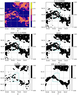Figure 7

The integral measure of geodynamic safety $\nu (w)$ (10) according to four specified features is shown in Figure 7a.

#### 2.2 Functional Clustering of the Integral Measure of Geodynamic Safety

The final measure of geodynamic safety $\nu$ (10) inherits to a certain extent stochasticity of the relief and fractures underlying it (Figure 7a). That's why, choosing a certain level $\alpha$, for example, $\alpha=0.45$, we see (Figure 7b) that the set of $\alpha$-stable nodes has a complex topology. This is related with the extreme heterogeneity of the geological environment. It is known that the most dangerous from the tectonic point of view are related areas often with a linearly elongated shape. Therefore, simplification is required, i.e. recognition of only massive areas with possible corrections of insignificant internal losses of $\alpha$-stability for final assessment of geodynamic safety. Simplification of the integral measure of geodynamic safety allows an expert to see visually and evaluate the main patterns in its distribution through the area, omitting insignificant details serving as background noises.

Use of traditional methods (ordinary averaging (Figure 7c), convolution with the Gauss core (Figure 7d) [Shapiro et al., 2001; Nixon et al., 2019], pyramidal smoothing (Figure 7e) [Smith, 1999]) for this purpose does not solve the problem. The required simplification is achieved using the FDPS algorithm. Figure 7f shows the result of using the FDPS algorithm with the selected density level for the measure given in Figure 7a. Figure 7f clearly demonstrates that the zone with a higher value $\nu$ intersects the underground research laboratory mine take in the direction from the southeast to the northwest.

### Conclusion

As a result of construction of the integral measure of geodynamic safety, it became possible to use the system analysis methods when assessing stability of structural and tectonic blocks of the Earth's crust for the urgent geoecological problem - ensuring safety of disposal of the high-level radioactive waste in geological formations. It should be noted that the results of using the algorithm as applied to the real-valued data of the Nizne-Kansk Massif are preliminary. The method requires to use a wider set of layers of analyzed data and needs to be improved.

In theoretical terms, continuation of researches related to the FDPS algorithm is seen by the authors:

• in automating selection of its parameters through optimization of the external quality functional simultaneously monitoring the massiveness of the obtained clusters and the "good" nodes of the required $\nu$-quality contained in them;
• in possible further clustering (uniting) of the $r$-connected components of its result, which will make it possible to distinguish more qualitatively the bases of the elevation on $X$ for the weight function $\nu$ and form the selection $B(X,\nu)$.

As to our problem, this will allow to identify the most stable structural blocks according to the values of the measure $\nu$.

Testing of the developed method and DMA algorithms based on several data layers for the northern part of the Nizne-Kansk Massif, where construction of the underground research laboratory is started at present to substantiate safety of deep HLRW disposal, and calculation of the geodynamic safety measure for the Yenisei area has shown their practical value and necessity of their further development, including for solving the geodynamic zoning problems [Gvishiani et al., 2019a].

An evident practical value of the method consists in the system step-by-step holistic analysis of diverse, multi-scale and multi-format layers of geological and geophysical information about the state of the structural-tectonic block, and, first of all, geomorphological, kinematic (determined based on the geodetic observations) and geophysical characteristics. A concept - a measure of activity of the dynamic indicator based on expert assessments of the behavior of geological and geophysical parameters in the vicinity of the grid nodes dividing the area into clusters is introduced for a formalized assessment of stability using the DMA methods. The cluster component of the DMA, based on the concept of density, allows to define strictly the concepts of condensation (dense subset), cluster (isolated condensations), and traces (linear condensations) for the multidimensional array. The FDPS algorithm was used to filter and arrange layers of geospatial data into homogeneous groups and separate dense homogeneous clusters that may be related to the deep zones of dynamic instability in the Earth's upper crust.

The preliminary data of the algorithm testing showed that the structural tectonic block, in which construction of a deep HLRW disposal site is planned, is located in a relatively stable zone. The FDPS algorithm can also be useful in planning comprehensive geophysical studies in the area of the underground research laboratory within the Nizne-Kansk Massif, as well as in solving other related problems in the sphere of geodynamics, geoecology and mining [Gvishiani et al., 2020].

#### Acknowledgments

The research was supported by Russian Science Foundation No. 18-17-00241 "Study of the rock massifs stability by system analysis of geodynamic processes for geoecologically safe underground of radioactive waste isolation".

### References

Anderson, E. B., S. V. Belov, E. N. Kamnev, et al. (2011) , Underground isolation of radioactive waste., 592 p. pp., Mining book, Moscow (in Russian).

Agayan, S., Sh. Bogoutdinov, R. Krasnoperov (2018) , Short introduction into DMA, Russian Journal of Earth Sciences, 18, no. 2, https://doi.org/10.2205/2018ES000618.

Belov, S. V., V. N. Morozov, V. N. Tatarinov, et al. (2007) , Study of the structure and geodynamic evolution of the Nizhnekansky Massif in connection with the disposal of highly radioactive waste, Geoecology, no. 2, p. 248–266 (in Russian).

Everitt, B. S. (1980) , , Cluster Analysis, p. 170 p., Halsted-Heinemann, London.

Gvishiani, A. D., B. A. Dzeboev, S. M. Agayan (2016) , FCAZm intelligent recognition system for locating areas prone to strong earthquakes in the Andean and Caucasian mountain belts, Izv., Phys. Solid Earth, 52, no. 4, p. 461–481, https://doi.org/10.1134/S1069351316040017 (in Russian).

Gvishiani, A. D., S. M. Agayan, B. A. Dzeboev, et al. (2017) , Recognition of strong earthquake–prone areas with a single learning class, Phys. Solid Earth, 474, no. 1, p. 546–551, https://doi.org/10.1134/S1028334X17050038 (in Russian).

Gvishiani, A. D., V. I. Kaftan, R. I. Krasnoperov, et al. (2019) , Geoinformatics and systems analysis in geophysics and geodynamics, Izvestiya. Physics of the Solid Earth, 55, no. 1, p. 33–49, https://doi.org/10.1134/S1069351319010038 (in Russian).

Gvishiani, A. D., S. M. Agayan, Sh. R. Bogoutdinov (2019) , Investigation of systems of real functions on two-dimensional grids using fuzzy sets, Chebyshevskii sbornik, 20, no. 1, p. 94–111, https://doi.org/10.22405/2226-8383-2019-20-1-94-111 (in Russian).

Gvishiani, A. D., V. N. Tatarinov, V. I. Kaftan, et al. (2020) , The velocities of modern horizontal movements of Earth crust in the South sector of Yenisei ridge according to GNSS observations, Doklady Earth Sciences, 493, no. 1, p. 73–77, https://doi.org/10.1134/S1028334X20070077 (in Russian).

Lobatskaya, R. M. (2005) , Neotectonic fault-block structure of junction of Siberian platform and West Siberian plate, Geology and Geophysics, 46, no. 2, p. 141–150 (in Russian).

Mikhailov, V. O., A. Galdeano, M. Diament, et al. (2003) , Application of artificial intelligence for Euler solutions clustering, Geophysics, 68, no. 1, p. 168–180.

Morozov, V. N., S. V. Belov, I. Yu. Kolesnikov, et al. (2008) , Possibilities of geodynamic zoning when choosing places for underground isolation of high-level radioactive waste on the example of the Nizhnekansky massif, Engineering ecology, no. 5, p. 17–25 (in Russian).

Nixon, M., A. Aguado (2019) , , Feature Extraction and Image Processing, p. 650p., Academic Press, USA.

Petukhov, I. M., I. M. Batugina (1999) , Geodynamic of the Earth Interior, 287 p. pp., Nedra Communications, Moscow (in Russian).

Shapiro, L. G., G. C. Stockman (2001) , , Computer Vision, p. 580 p., Prentence Hall, NJ.

Smith, S. W. (1999) , , The Scientist and Engineer's Guide to Digital Signal Processing, p. 664 p., California Technical Publishing, San Diego, California.

Soloviev, A., A. Chulliat, S. Bogoutdinov, et al. (2012) , Automated recognition of spikes in 1 Hz data recorded at the Easter Island magnetic observatory, Earth Planets Space, 64, no. 9, p. 743–752, https://doi.org/10.5047/eps.2012.03.004.

Tatarinov, V. N., V. N. Morozov, I. Yu. Kolesnikov, et al. (2014) , Stability of the geological environment as the basis for safe underground isolation of radioactive waste and spent nuclear fuel, Reliability and Safety of Power Engineering, no. 1 (24), p. 25–29 (in Russian).

Tatarinov, V. N., V. N. Morozov, I. Yu. Kolesnikov, et al. (2014) , Kinematic method of geodynamic zoning in the design of underground mining, Bezopasnost zhiznedeyatelnosti, no. 7, p. 8–11 (in Russian).

Tou, J. T., R. C. Gonzalez (1974) , , Pattern Recognition Principles, p. 378 p., Addison-Wesley Publishing Company, USA.

Trofimov, V. T., N. S. Gerasimova, N. S. Krasilova (1994) , Stability of the geological environment and factors that determine it, Geoecology, no. 2, p. 18–28 (in Russian).

Zadeh, Lotfi A. (1996) , , Fuzzy Sets, Fuzzy Logic, and Fuzzy Systems, p. 801 p., World Scientific Publishing Co., Inc., NJ.

Zlotnicki, J., J.-L. LeMouel, A. Gvishiani, et al. (2005) , Automatic fuzzy-logic recognition of anomalous activity on long geophysical records. Application to electric signals associated with the volcanic activity of la Fournaise volcano (Reunion Island), Earth and Planetary Science Letters, 234, p. 261–278.

Received 19 April 2020; accepted 10 September 2020; published 18 December 2020.Citation: Agayan S. M., V. N. Tatarinov, A. D. Gvishiani, Sh. R. Bogoutdinov, I. O. Belov (2020), FDPS algorithm in stability assessment of the Earth's crust structural tectonic blocks, Russ. J. Earth Sci., 20, ES6014, doi:10.2205/2020ES000752.

Generated from LaTeX source by ELXfinal, v.2.0 software package.